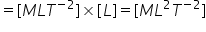# Work

Work:

Work is said to be done when a force applied on the body displaces the body through a certain distance in the direction of force.

Let a constant force F be applied on the body such that it makes an angle θ  with the horizontal and body is displaced through a distance s along horizontal direction.

(i) F cosθ   acts in the direction of displacement of the body.

(ii) F sinθ  acts in the perpendicular direction of displacement of the body.

Since body is being displaced in the direction of Fcosθ , no work is done by Fsinθ and therefore work done by the force in displacing the body through a distance s is given by

W= F cosθ sWork Done by any  force is equal to the scalar or dot product of the force and the displacement of the body.

Important Points

1. Work is a method by which energy is transferred from one object to another object by the application of force.
2. Positive work means energy is transferred to the object by the application of force.
3. Negative Work means energy is being from the object by the application of force.
4. Zero work done means there is no exchange of energy between two objects on the application of force.
5. In dW= F.ds  , dW is infinitely small Work done by the Object during the displacement ds of point of application of force with respect to observer.
6. ds is small displacement of the point of application of force with respect to Observer.In general it is taken with respect to Ground.
7. Work is a Relative Quantity.
8. While calculating Work Done think a)by which force work is to be calculated     b)what is the displacement of point of application of force.  c) Force is constant or variable  d) angle is constant or variable .

Example :

A man is climbing up the stairs , then work done by the normal force on the man is Zero.

Dimension and Units of Work.

Dimension : work =  Force x displacement

[W] = [Force] x [Displacement]Units :

Joule (S.I.): Work done is said to be one Joule, when 1 Newton force displaces a body through 1 meter  in its own direction.

W = F.s

1 Joule = 1 Newton  x 1 metre

Erg (C.G.S.) : Work done is said to be one erg when 1 dyne force displaces a body through 1 cm in its own direction.

W = F s

1 Erg = 1 Dyne x 1 cm

#### Relation between Joule and erg

1 Joule =  107Erg

The unit of work is joule. One joule is defined as the work done by a force of one newton when its point of application moves by one metre along the line of action of the force.

Related Keywords